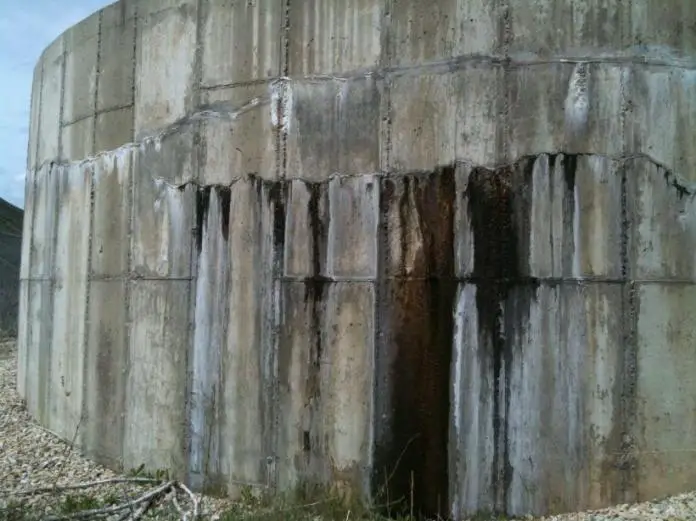# Cracking Due to Edge Restraint and Early Thermal Effects in ConcreteCrack width is calculated by multiplying the crack inducing strain by the crack spacing (i.e. the movement over a length equal to the crack spacing). Crack inducing strain εcr is calculated based on whether the element is subjected to edge restraint (which can be early or long term thermal effects), end restraint, and flexure/direct tension. In this article, we are going to shown how to calculate the crack width of a structural element subjected to edge restraint and early thermal cracking.

Edge restraint occurs where the young concrete section (say a wall) is cast on a hardened concrete base. This means that restriction is only in one direction, and there is interaction between the old and new concrete in terms of distribution of cracks. Edge restraint is different from end restraint because the crack width is a function of restrained strain rather than the tensile capacity of the concrete.

At the early age of freshly poured concrete (within 3 days), the crack inducing strain due to edge restraint in concrete element is given in equation (3.6) of CIRIA C660 which is shown below.

εcr = K[αcT1 + εca] R1 – 0.5 εctu

where;
K = allowance for creep
= 0.65 when R is calculated using CIRIA C660
= 1.0 when R is calculated using BS EN 1992-3.

αc = coefficient of thermal expansion
T1 = difference between the peak temperature of concrete during hydration and ambient temperature °C (See Table 1).
εca = autogenous shrinkage strain – value for early age (3 days, see Table 2)
R1 = restraint factor from Figure L1 of BS EN 1992-3 for the short-term situation
εctu = tensile strain capacity of the concrete (see Table 3)

Table 1: Typical values of difference between the peak temperature of concrete during hydration and ambient temperature (Narayanan and Goodchild, 2012)

Table 2: Typical Values of Autogenous Shrinkage Strain

Table 3: Values of Tensile Strain Capacity of Concrete (CIRIA C660)

Calculation Example

Calculate the early thermal crack width of concrete wall cast in Nigeria with the following data;

Thickness of wall = 400 mm
Reinforcement provided = H12@150mm c/c on both faces
Concrete cover = 50 mm
Type of restriant = Edge restraint
Type of coarse aggregate = Granite

Solution

Crack width wk = sr,max εcr

The maximum crack spacing sr,max = 3.4c + 0.425 (k1 k2ϕ/ρp,eff)

To see the definition of these terms, see the post below;

Crack width and crack spacing calculation in concrete

c = 50 mm to outer face
k1 = 0.8
k2 = 1.0
ϕ = 12 mm
ρp,eff  = 754/{1000 × min[400/2; 2.5 × (50 + 12/2)]}
ρp,eff  = 754/(1000 × 140) = 0.00538

sr,max = 3.4 × 50 + 0.425 (0.8 × 1.0 × 12 / 0.00538) = 170 + 758.36 = 928.36 mm

Early age crack-inducing strain, εcr = K[αcT1 + εca] R – 0.5 εctu

Using CIRIA C660 the following parameters can be determined;

K = 0.65
αc = 10 × 10–6 (granite coarse aggregate, see coefficient of thermal expansion of concrete)
T1 = Using 35 °C (for a 400 mm thick wall cast in Nigeria, assuming Class N cement, 18 mm marine plywood, concrete grade C30/37 and cement content not less than 360 kg/m3, see Table 1)
εca (autogenous shrinkage strain for grade 30/37 concrete at 3 days) = 15 × 10–6 (see Table 2)
R = Restraint factor Rj =  1/(1 + EnAn/EoAo)Where;

En and Eo are the elastic modulus of new and old concrete respectively
Assume En/Eo = 0.80 (CIRIA C660)
An and Ao are the areas of new and old concrete respectively (use An/Ao = hn/2ho assuming that the wall is cast remote from the edge of slab) = 0.4/2(0.4) = 0.5

R = 1/[1 + (0.8 × 0.5)] = 0.714

εctu = 76 × 10–6 (for early age thermal cracking, see Table 3)

εcr = K[αcT1 + εca] R – 0.5 εctu = 0.65 [(10 × 10–6 × 35) + 15 × 10–6] 0.714 – 0.5(76 × 10–6) = 1.3139 × 10–4

Therefore the early age crack width = wk = sr,max εcr = 928.36 x 1.3139 × 10–4 = 0.121 mm

Since this crack width is less than 0.2mm, the early crack width can be considered acceptable for water retaining structures.